# Midterm Exam 1 14 12 10 8 6

• Slides: 15
Download presentation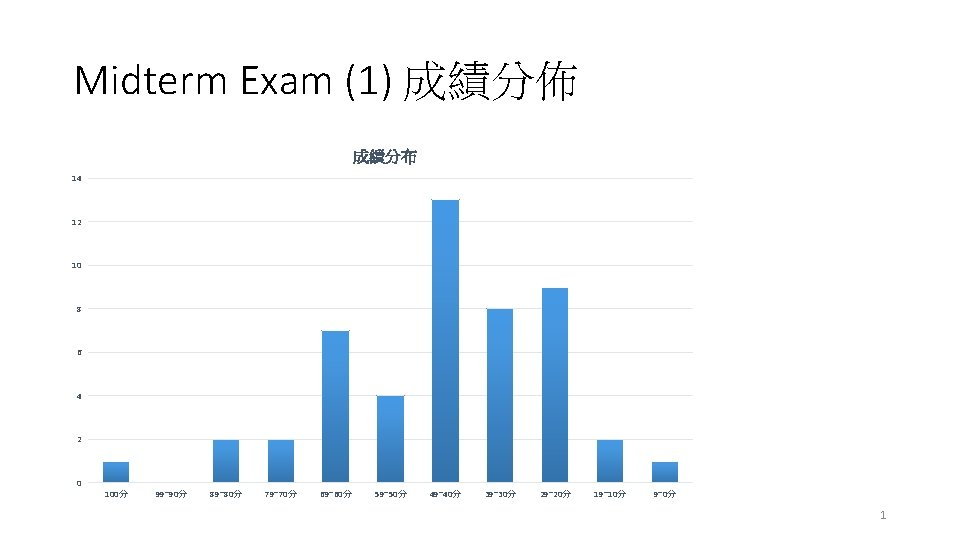Midterm Exam (1) 成績分佈 成績分布 14 12 10 8 6 4 2 0 100分 99~90分 89~80分 79~70分 69~60分 59~50分 49~40分 39~30分 29~20分 19~10分 9~0分 1Chapter 7 Arrays and Vectors 3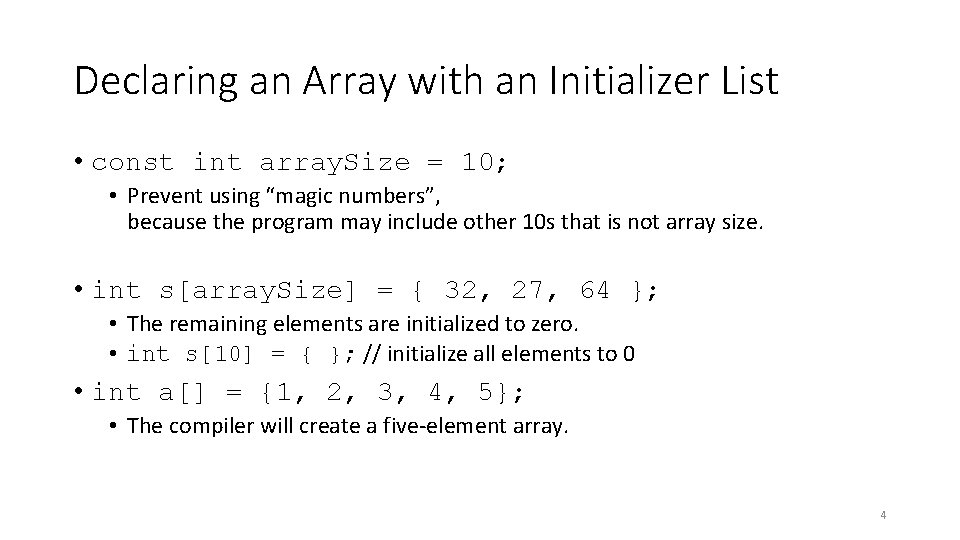Declaring an Array with an Initializer List • const int array. Size = 10; • Prevent using “magic numbers”, because the program may include other 10 s that is not array size. • int s[array. Size] = { 32, 27, 64 }; • The remaining elements are initialized to zero. • int s = { }; // initialize all elements to 0 • int a[] = {1, 2, 3, 4, 5}; • The compiler will create a five-element array. 4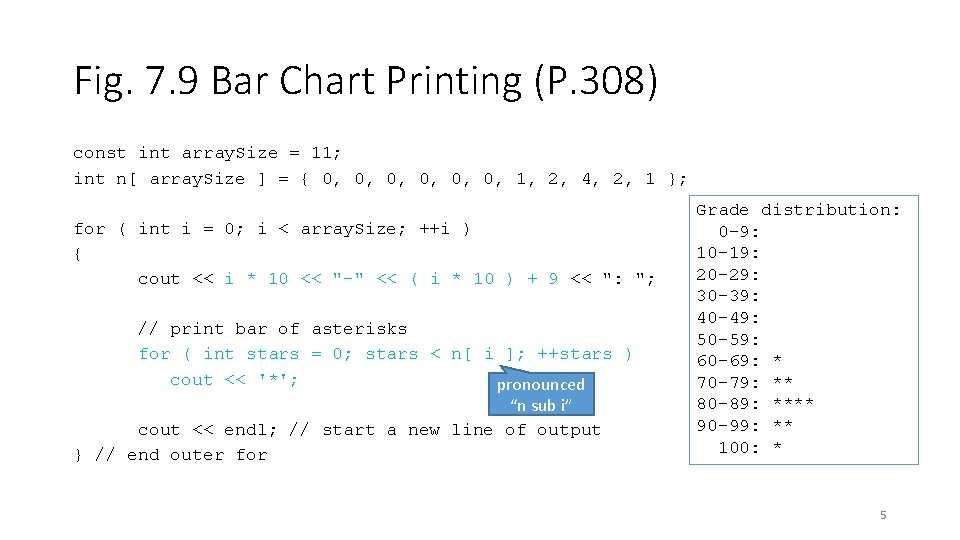Fig. 7. 9 Bar Chart Printing (P. 308) const int array. Size = 11; int n[ array. Size ] = { 0, 0, 0, 1, 2, 4, 2, 1 }; for ( int i = 0; i < array. Size; ++i ) { cout << i * 10 << "-" << ( i * 10 ) + 9 << ": "; // print bar of asterisks for ( int stars = 0; stars < n[ i ]; ++stars ) cout << '*'; pronounced “n sub i” cout << endl; // start a new line of output } // end outer for Grade distribution: 0 -9: 10 -19: 20 -29: 30 -39: 40 -49: 50 -59: 60 -69: * 70 -79: ** 80 -89: **** 90 -99: ** 100: * 5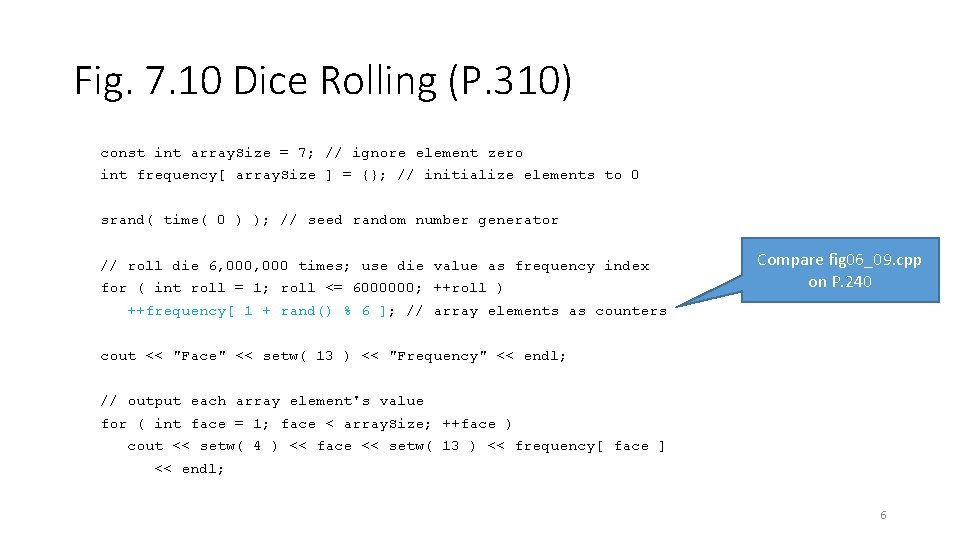Fig. 7. 10 Dice Rolling (P. 310) const int array. Size = 7; // ignore element zero int frequency[ array. Size ] = {}; // initialize elements to 0 srand( time( 0 ) ); // seed random number generator // roll die 6, 000 times; use die value as frequency index for ( int roll = 1; roll <= 6000000; ++roll ) Compare fig 06_09. cpp on P. 240 ++frequency[ 1 + rand() % 6 ]; // array elements as counters cout << "Face" << setw( 13 ) << "Frequency" << endl; // output each array element's value for ( int face = 1; face < array. Size; ++face ) cout << setw( 4 ) << face << setw( 13 ) << frequency[ face ] << endl; 6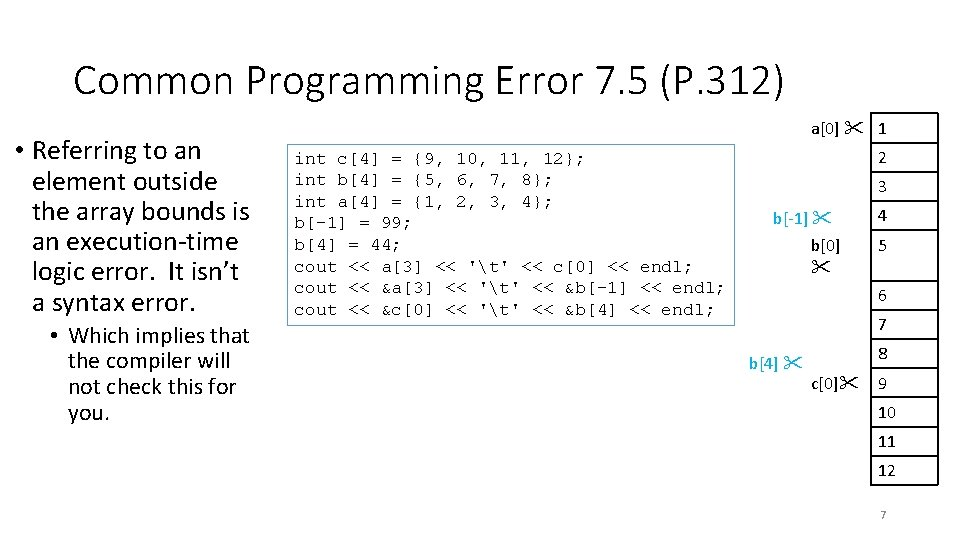Common Programming Error 7. 5 (P. 312) • Referring to an element outside the array bounds is an execution-time logic error. It isn’t a syntax error. • Which implies that the compiler will not check this for you. a 1 int c = {9, 10, 11, 12}; int b = {5, 6, 7, 8}; int a = {1, 2, 3, 4}; b[-1] = 99; b = 44; cout << a << 't' << c << endl; cout << &a << 't' << &b[-1] << endl; cout << &c << 't' << &b << endl; 2 3 b[-1] b 4 5 6 7 b 8 c 9 10 11 12 7Fig. 7. 13 Passing Arrays to a Function (P. 316) void modify. Array( int [], int ); Even if you include the array size, it will be ignored by the compiler. void modify. Array( int b[], int size. Of. Array ) { // multiply each array element by 2 for ( int k = 0; k < size. Of. Array; ++k ) b[ k ] *= 2; } // end function modify. Array 8Fig. 7. 14 const parameter (P. 318) void try. To. Modify. Array( const int b[] ) { b[ 0 ] /= 2; } compilation error if you modify elements in this array 9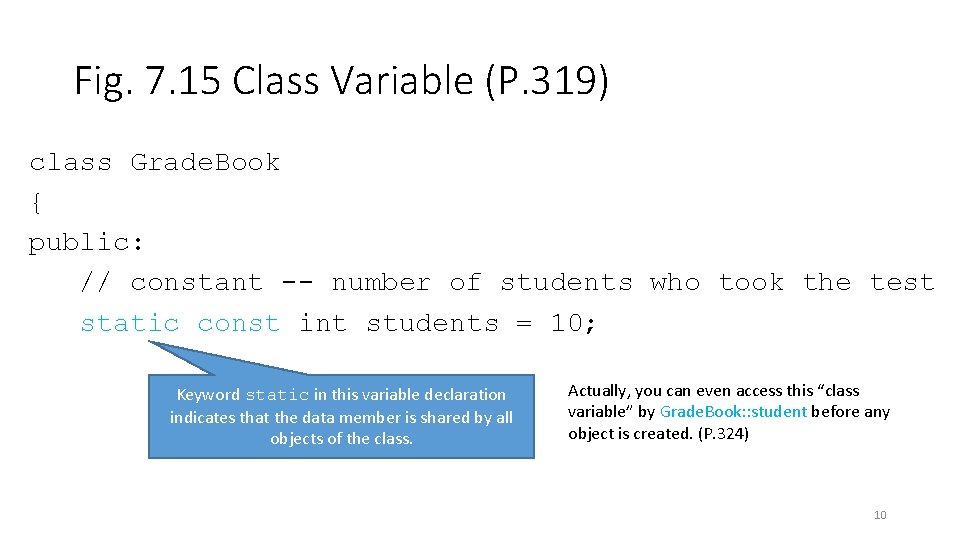Fig. 7. 15 Class Variable (P. 319) class Grade. Book { public: // constant -- number of students who took the test static const int students = 10; Keyword static in this variable declaration indicates that the data member is shared by all objects of the class. Actually, you can even access this “class variable” by Grade. Book: : student before any object is created. (P. 324) 10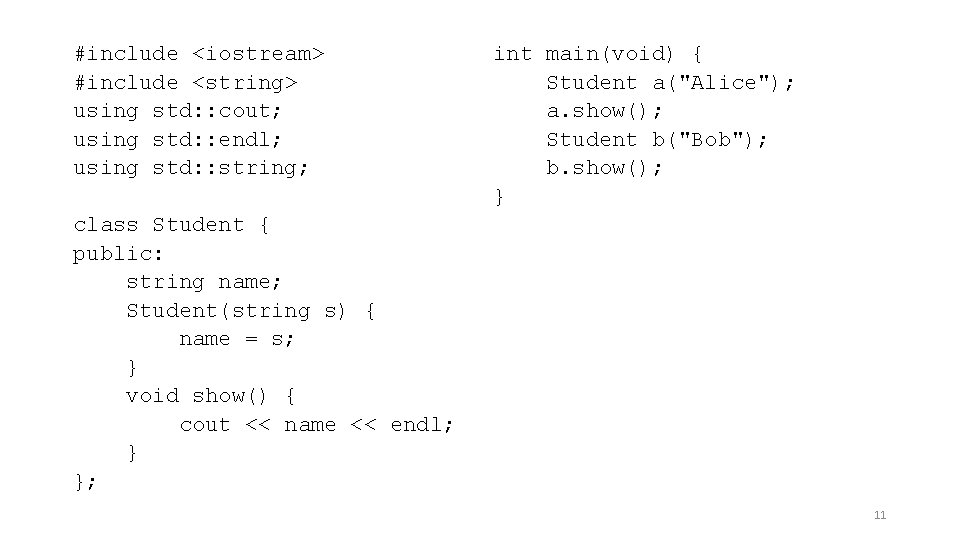#include #include using std: : cout; using std: : endl; using std: : string; int main(void) { Student a("Alice"); a. show(); Student b("Bob"); b. show(); } class Student { public: string name; Student(string s) { name = s; } void show() { cout << name << endl; } }; 11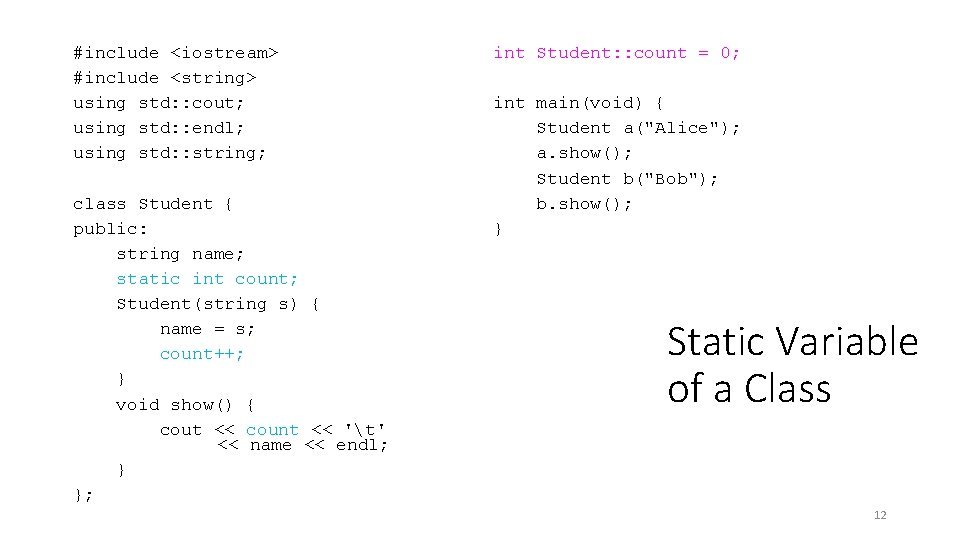#include #include using std: : cout; using std: : endl; using std: : string; class Student { public: string name; static int count; Student(string s) { name = s; count++; } void show() { cout << count << 't' << name << endl; } }; int Student: : count = 0; int main(void) { Student a("Alice"); a. show(); Student b("Bob"); b. show(); } Static Variable of a Class 12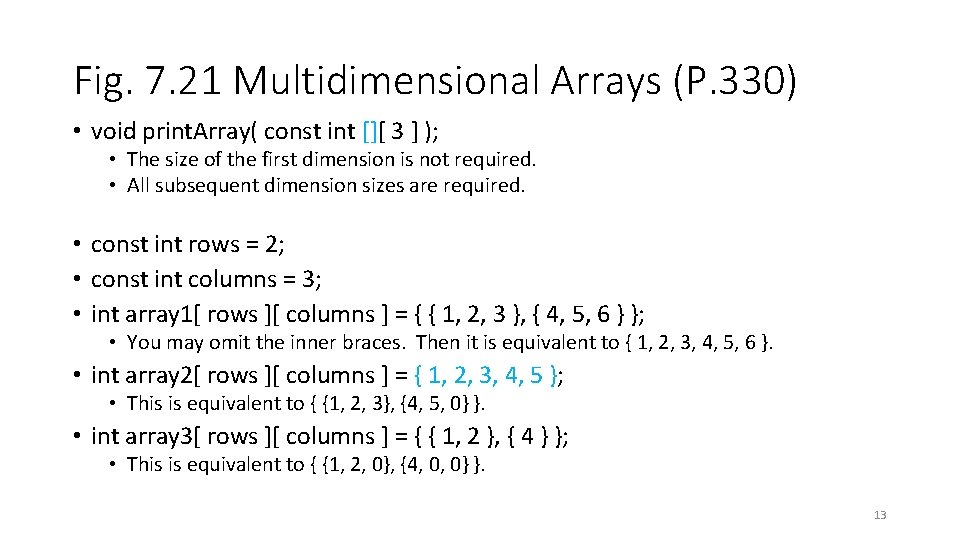Fig. 7. 21 Multidimensional Arrays (P. 330) • void print. Array( const int [][ 3 ] ); • The size of the first dimension is not required. • All subsequent dimension sizes are required. • const int rows = 2; • const int columns = 3; • int array 1[ rows ][ columns ] = { { 1, 2, 3 }, { 4, 5, 6 } }; • You may omit the inner braces. Then it is equivalent to { 1, 2, 3, 4, 5, 6 }. • int array 2[ rows ][ columns ] = { 1, 2, 3, 4, 5 }; • This is equivalent to { {1, 2, 3}, {4, 5, 0} }. • int array 3[ rows ][ columns ] = { { 1, 2 }, { 4 } }; • This is equivalent to { {1, 2, 0}, {4, 0, 0} }. 13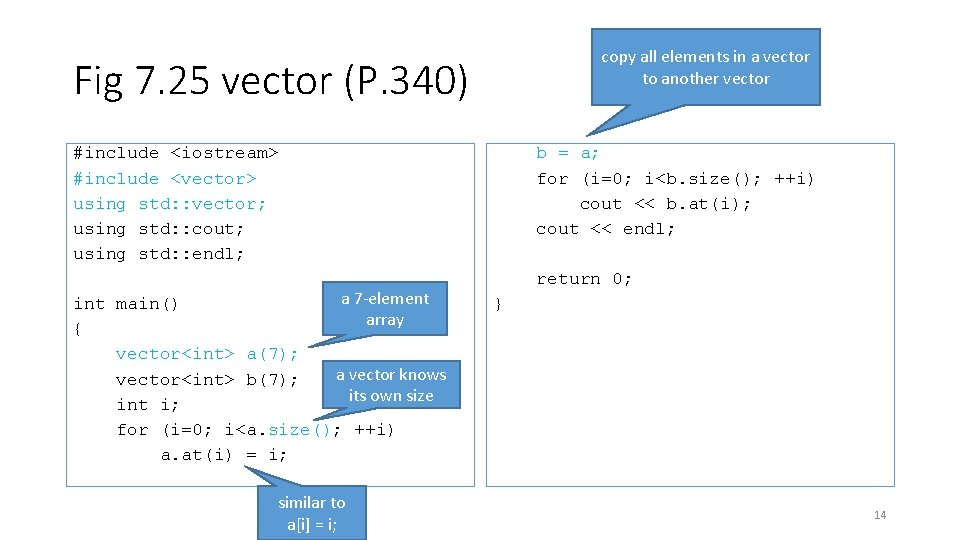copy all elements in a vector to another vector Fig 7. 25 vector (P. 340) #include #include using std: : vector; using std: : cout; using std: : endl; a 7 -element int main() array { vector a(7); a vector knows vector b(7); its own size int i; for (i=0; i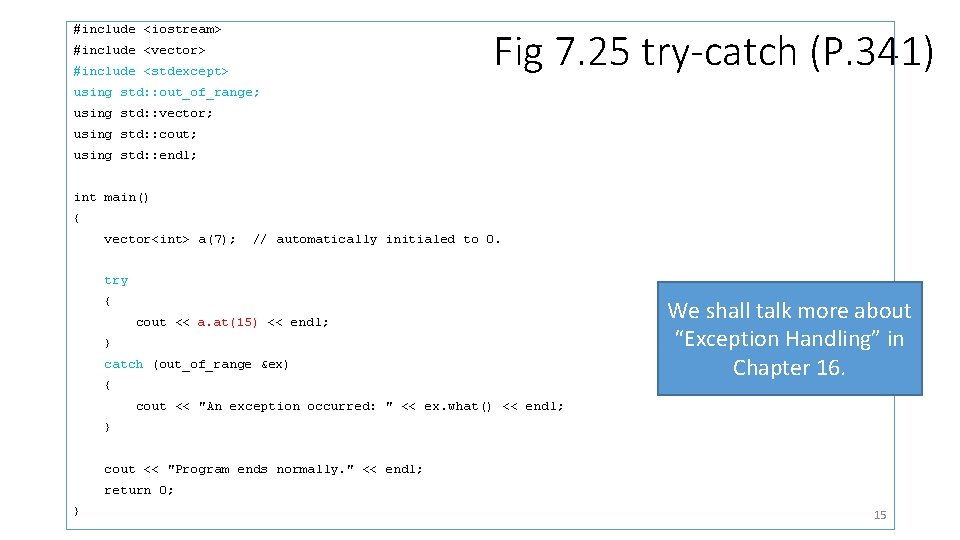#include Fig 7. 25 try-catch (P. 341) #include #include using std: : out_of_range; using std: : vector; using std: : cout; using std: : endl; int main() { vector a(7); // automatically initialed to 0. try { cout << a. at(15) << endl; } catch (out_of_range &ex) { We shall talk more about “Exception Handling” in Chapter 16. cout << "An exception occurred: " << ex. what() << endl; } cout << "Program ends normally. " << endl; return 0; } 15